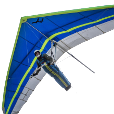chain co linear-ish splines

Recommended PostsHi guys. I have bunch of splines. While I manage to retrieve the coordinates of endpoints, divide the purple parts (so no part of purple section are longer than 300), retrieve the coordinates of the division, since panels are very organic shaped I cannot solely rely on the coordinates to figure out the following:  find out which splines are "colinear-ish" (shown in purple in the sample dwg). I tried use "join" to regroup chains of them but join fails, some portions get discarded from operation. (fuzz factor on endpoints or not perfectly co-linear). When it doesn't the result isn't what I need, as non colinear-ish (shown in white) sometimes get joined too.  Ultimately what i'm trying to do is to re trieve the lists of coordinates of the points shown by cyan leaders in chain order, from one end to the other, on both sides.

new block - Copie.dwg

Share on other sitesI do not get it clear . Please enlighten me.

Share on other sitesHow about exploding the cyan leaders and then add a 3dpoly using osnap end from arrowhead tip to arrowhead tip.  The 3dpoly will have the coordinates you need in order. Then use list and copy paste to transfer and format the coordinates in Excel like this:

x                     y                     z
159702.25    17193.02     350.19
159501.66    17320.73     184.69
159313.37    17440.6         0
159144.51    17548.11    -209.21
159010.14    17633.65    -450.62
158891.01    17709.49    -534.15
158706.44    17827        -527
158521.87    17944.5        -519.85
158337.3     18062.01    -512.7

Share on other sitesMaybe this helps:

```(defun KGA_Geom_VectorScale (vec scl)
(mapcar '(lambda (a) (* a (float scl))) vec)
)

(defun KGA_Geom_VectorUnit (vec / mag)
(if (/= 0.0 (setq mag (distance '(0.0 0.0 0.0) vec)))
(KGA_Geom_VectorScale vec (/ 1.0 mag))
)
)

; (CurveSmoothConnect_P (car (entsel)) (car (entsel)))
(defun CurveSmoothConnect_P (objA objB / fuzz staA endA staB endB vecStaA vecEndA vecStaB vecEndB)
(setq fuzz 1e-3)
(setq staA (vlax-curve-getstartpoint objA))
(setq endA (vlax-curve-getendpoint objA))
(setq staB (vlax-curve-getstartpoint objB))
(setq endB (vlax-curve-getendpoint objB))
(setq vecStaA (KGA_Geom_VectorUnit (vlax-curve-getfirstderiv objA (vlax-curve-getstartparam objA))))
(setq vecEndA (KGA_Geom_VectorUnit (vlax-curve-getfirstderiv objA (vlax-curve-getendparam objA))))
(setq vecStaB (KGA_Geom_VectorUnit (vlax-curve-getfirstderiv objB (vlax-curve-getstartparam objB))))
(setq vecEndB (KGA_Geom_VectorUnit (vlax-curve-getfirstderiv objB (vlax-curve-getendparam objB))))
(or
(and
(equal staA endB fuzz)
(equal vecStaA vecEndB fuzz)
)
(and
(equal staA staB fuzz)
(equal vecStaA (mapcar '* '(-1.0 -1.0 -1.0) vecStaB) fuzz)
)
(and
(equal endA staB fuzz)
(equal vecEndA vecStaB fuzz)
)
(and
(equal endA endB fuzz)
(equal vecEndA (mapcar '* '(-1.0 -1.0 -1.0) vecEndB) fuzz)
)
)
)```

•1

Share on other sites@devitg13 hours ago, lrm said:

I added the leaders for clarity purpose. They are not in the model, only the splines are.

I had 2 light-bulb moment yesterday, 2 different ways of solving this... @Roy_043 I'm learning curve related maths and functions as I use them, (not the easiest thing using examples in Adesk help. ie.: vlax-curve-getFirstDeriv; "Returns the first derivative of a curve at the specified location" doesn't give much clue about what a derivative is, and examples such as yours in a specific context is a great academic value.) That vlax-curve-getfirstderiv is definitely a 3rd approach. Very helpful indeed. Thank you very much!

Join the conversation

You can post now and register later. If you have an account, sign in now to post with your account.
Note: Your post will require moderator approval before it will be visible.×   Pasted as rich text.   Paste as plain text instead

Only 75 emoji are allowed.